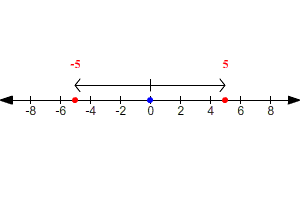# Opposites

The opposite of a number is its additive inverse. The sum of a number and its opposite is zero. (This is sometimes called the property of opposites ).

$a+\left(-a\right)=0$

Examples:

$\begin{array}{l}-999+999=0\\ \frac{1}{3}+\left(-\frac{1}{3}\right)=0\\ 1+\left(-1\right)=0\end{array}$

So, the opposite of $-999$ is $999$ ; the opposite of $\frac{1}{3}$ is $-\frac{1}{3}$ ; the opposite of $1$ is $-1$ .

You can also think of the opposite of a number as its reflection around $0$ on the number line.